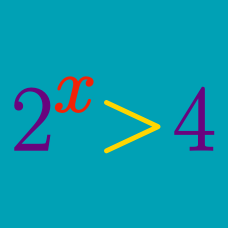Algebra

# Exponential Inequalities - Similar Base

What is the range of $x$ that satisfies the inequality $4^x < 16^{6}?$

What is the range of $x$ that satisfies the inequality $\frac{3^{x^2}}{9^{2x}}<1?$

What is the range of $x$ that satisfies the inequality $4^x < 2^{x+6}?$

What is the range of $x$ that satisfies the inequality $4^{x-4} > \frac{64^x}{4^{8}}?$

What is the range of $x$ that satisfies the inequality $\frac{1}{3} < 9^{\frac{1}{2}x+3} < \sqrt{3^4} ?$

×